# Preface

This chapter is supposed to help you with developing very important skills---plotting. Fortunately, Mathematica has a tremendous arsenal of tools to accomplish almost any plotting task. This chapter remembers that the presented material is for beginners and more advanced codes could be found elsewhere, in particular, in the second part of this tutorial.

# Plotting

One of the best characteristics of Mathematica is its plotting ability. It is very easy to visualize diversity of outputs generated by Mathematica. This computer algebra system has a variety of two-dimensional plotting commands:

In addition, Mark A. Caprio from the University of Notre Dame prepared a package SciDraw for preparing publication-quality scientific figures with Mathematica. SciDraw provides both a framework for structuring figures and tools for generating their content. SciDraw helps with generating figures involving mathematical plots, data plots, and diagrams.

The basic plotting command, Plot, is simple to use. To make a plot, it is necessary to define the independent variable that you are graphing with respect to. Mathematica automatically adjusts the range over which you are graphing the function.

Plot[2*Sin[3*x]-2*Cos[x], {x,0,2*Pi}]In the above code, we use a natural domain for the independent variable to be $$[0,2\pi ] .$$ In general, the domain of the independent variable is usually chosen based on a particular interest. As with all Mathematica commands, the output can be highly customized by using options. The great benefit of the option method is that the order in which the options are placed does not matter. To find the options corresponding to Plot, type in
Options[Plot]
Many of these options will be discussed in the tutorial later. Here we present one of them
Plot[{Cos@x, ArcCos@x}, {x, -Pi, Pi}, PlotStyle -> Thick]
In this command sequence, the independent variable is x and the range is 0 to $$2\pi .$$ For Plot, after entering the function that you wish to graph, you separate the equation and add {independent variable, lower bound, upper bound}.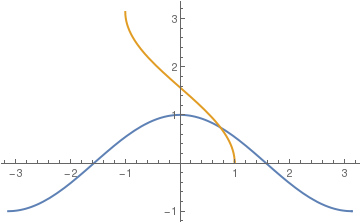In this example, we are just plotting a function using Mathematica default capabilities. Plot uses several internal algorithms that one should become familiar with. First of all, Plot tries to determine the region of visual interest and restrict the plotting range to that region. This can be overridden by setting PlotRange to All or to a specific interval for the vertical range (and also the horizontal range if desired). A similar graph can also be obtained with the following script:
Plot[3 Cos[x] - 2 Sin[3 x], {x, -3*Pi, 3*Pi}, PlotRange -> {-5, 6}, Frame -> True, FrameTicks -> {{Automatic, None}, {Range[-3 Pi, 3 Pi, Pi], None}}, Axes -> None, PlotStyle -> {Thick, Blue}]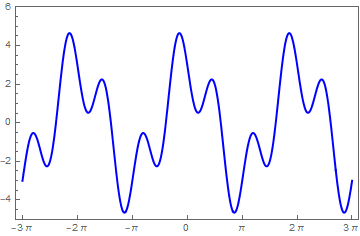As most computer systems, Mathematica can produce not only graphics but also sound. Mathematica treats graphics and sound in a closely analogous way, using command Play. For instance, the previous function can be used to play, on a suitable computer system, a pure tone with a frequency of 440/2π hertz for one second.

Play[2*Sin[3*x*440] - 2*Cos[x*440], {x, 0, 1}]

For multiple plots, use either command Show or you can use {} with commas. Show can be used to change the options of an existing graphic or to combine multiple graphics.

Plot[{2 Sin[3 x] - 2 Cos[x], Sin[x] - 1/3 Cos[3 x]}, {x, 0, 3}, PlotStyle -> Thick]Show command can be used to adjust the Background option of an existing graphic
g1 = Plot[Sin[x] - 1/3 Cos[3 x], {x, -1, 3}, PlotStyle -> Thick]
Show[{g1, Graphics[Circle[]]}, Background -> Yellow, AspectRatio -> Automatic]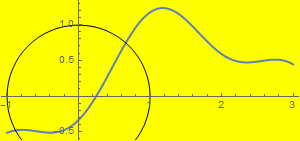Example: Plot sine function downward:

data1 = Table[{x, Sin[x]}, {x, -5, 5, 0.1}];
ListLinePlot[data1, PlotRange -> All];
ticks = Table[{-x, x}, {x, -5, 5, .2}];
ListLinePlot[{#, -#2} & @@@ data1, PlotRange -> {All, 1}, Ticks -> {All, ticks}, Axes -> True, PlotStyle -> Thick]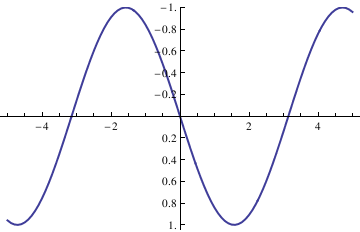data1 = Table[{x, Sin[x]}, {x, -5, 5, 0.1}];
ListLinePlot[data1, PlotRange -> All];
ticks = Table[{-x, x}, {x, -5, 5, .2}];
ListLinePlot[{#, -#2} & @@@ data1, PlotRange -> {-1.3, 1.3},
Ticks -> {All, ticks}, Frame -> False, PlotRange -> All,
Epilog -> {Text["x", {5.5, 0}], Text["y", {0, -1.4}]},
PlotRangeClipping -> False, ImagePadding -> {{20, 20}, {20, 20}}]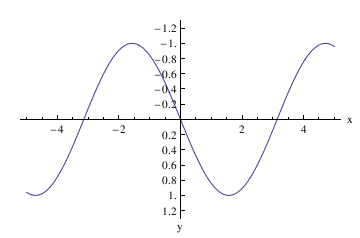Now plot with arrows, but without units:

axes[x_, y_, f_, a_] :=
Arrow[{{0, 0}, #}] & /@ {{x, 0}, {0, y}}, {Text[
Style["x", FontSize -> Scaled[f]], {0.95*x, 0.1*y}],
Text[Style["y", FontSize -> Scaled[f]], {0.1 x, 1*y}]}]]

data1 = Table[{x, Sin[x]}, {x, 0, 5, 0.1}];
ListLinePlot[data1, PlotRange -> All];
ticks = Table[{-x, x}, {x, -5, 5, .2}];
Show[ListLinePlot[{#, -#2} & @@@ data1, PlotRange -> {-1.3, 0},
Ticks -> {None, None}, Frame -> False, PlotRange -> All,
PlotRangeClipping -> False, ImagePadding -> {{20, 20}, {20, 20}}],
axes[5.3, -1.33, .06, .05], Axes -> False]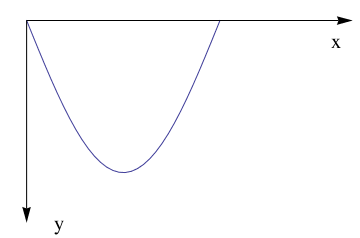Example: A Tractrix (from the Latin verb "trahere" -- pull, drag; plural: tractrices) is the curve along which an object moves, under the influence of friction, when pulled on a horizontal plane by a line segment attached to a tractor (pulling) point that moves at a right angle to the initial line between the object and the puller at an infinitesimal speed. By associating the object with a dog, the string with a leash, and the pull along a horizontal line with the dog's master, the curve has the descriptive name hundkurve (dog curve) in German. It is therefore a curve of pursuit. It was first introduced by Claude Perrault (1613--1688) in 1670. Trained as a physician, Claude was invited in 1666 to become a founding member of the French Academie des Sciences, where he earned a reputation as an anatomist. The first known solution was given by Christiaan Huygens (1692), who also named the curve the tractrix. Its parametric equation is

$x = a\, \sin (t), \qquad y = a\left( \cos (t) + \ln \left(\tan (t/2)\right) \right) .$

To plot tractrix curve, we use the following code which utilizes the Manipulate function:
tractrix[a_][t_] := a*{Sin[t], Cos[t] + Log[Tan[t/2]]};
Manipulate[
ParametricPlot[tractrix[a][t] // Evaluate, {t, 0, .99*\[Pi]},
PlotRange -> {0, 7}], {a, 1, 6}]
Export["tractrix1.gif",%]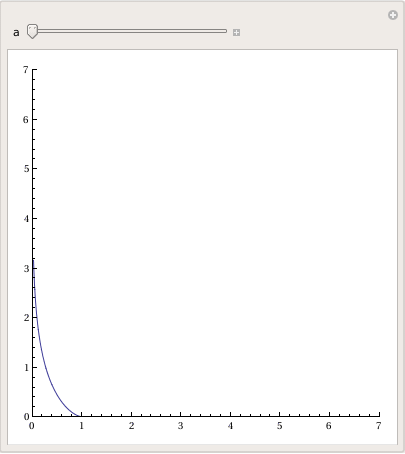Manipulate[
Plot[y'[x] = -Sqrt[a^2 - x^2]/x, {x, 0, 20},
PlotRange -> {-10, 10}], {a, 0, 20}]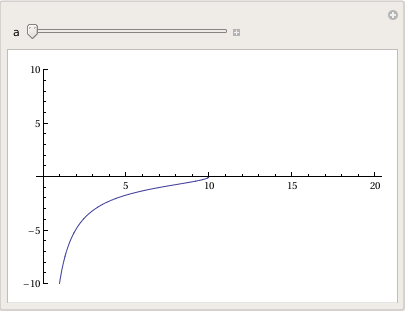sol = DSolve[{y'[x] == -Sqrt[a^2 - x^2]/x, y[a] == 0}, y, x]
Out= {{y -> Function[{x}, -Sqrt[a^2 - x^2] + a Log[a] - a Log[a^2] -
a Log[x] + a Log[a^2 + a Sqrt[a^2 - x^2]]]}}

Manipulate[
Plot[-Sqrt[a^2 - x^2] + a Log[a] - a Log[a^2] - a Log[x] + a Log[a^2 + a Sqrt[a^2 - x^2]], {x, 0, 20}, PlotRange -> All], {a, 1, 20}]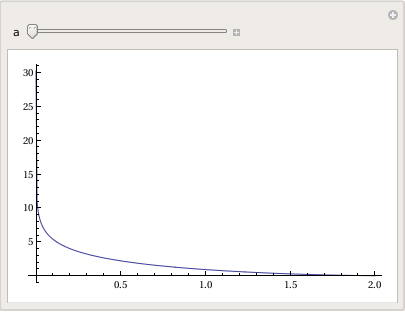Plotting dots can be acomplished with a special subroutine:
DotPlot[data_] := Module[{m = Tally[Sort[data]]}, ListPlot[Flatten[Table[{#1, n}, {n, #2}]& @@@ m, 1], Ticks -> {Automatic, Range[0, Max[m[[All, 2]]]]}] ]

1. Visualization Mathematica Tutorial by John Boccio
2. Plotting with Mathematica
3. 2D Graphs by Michael A. Morrison
4. Color Function by B G Higgins